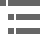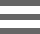## Microscope Conjugate Field PlanesThe geometrical relationship between image planes in the optical microscope is explored in this tutorial. In all of the imaging steps, with the exception of Image Plane (3'), the image is real and inverted.

The tutorial initializes with the Object Height slider set to the largest available value. The microscope optical tube length, which is the distance between the objective rear focal point (F') and the intermediate image plane, is indicated in the tutorial diagram. Light focused by the objective produces an image at I(3) (the intermediate image) that is further magnified by the eyepiece to produce an image on the retina at I(4). When the microscope eyepiece is used for direct viewing rather than for projection, the image at I(3') is not real but virtual and is not inverted relative to the intermediate image. The human eye will not perceive the image on the retina as inverted, even though the image is inverted in relation to the intermediate image and the virtual image.

The total magnification of the microscope can be determined by considering properties of the objective and eyepieces. Objectives are corrected for a particular projection distance, which is specific to the magnification and is approximately equal to the optical tube length. In a fixed tube length microscope, this projection distance is about 160 millimeters. Therefore, a 8-millimeter focal length objective would have a lateral magnification of about 20x (160/8) with a corresponding longitudinal magnification of 400x (20 × 20).

For visual observation, the eyepiece magnification is assumed to be unity when a specimen (or image) is placed at a distance of 250 millimeters from the eye of the observer. In this regard, an eyepiece having a focal length of 25 millimeters would have a magnification value of 10x (250/25). The total microscope magnification for visual observation is computed by taking the product of the objective and eyepiece magnifications. For the objective and eyepiece just described, the total lateral magnification would be about 200x (10x eyepiece multiplied by the 20x objective).

### Contributing Authors

John C. Long and Michael W. Davidson - National High Magnetic Field Laboratory, 1800 East Paul Dirac Dr., The Florida State University, Tallahassee, Florida, 32310.

# Microscopy U - The source for microscopy education•••